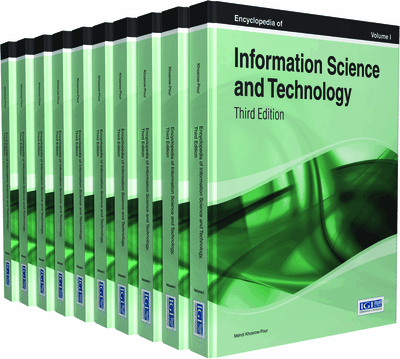# Current Quantum Information Science and Technology

Göran Pulkkis (Arcada University of Applied Sciences, Finland) and Kaj J. Grahn (Arcada University of Applied Sciences, Finland)
DOI: 10.4018/978-1-4666-5888-2.ch420

Top

## Information Representation With Quantum States

Quantum states are energy levels of molecules, atoms, and photons. Two quantum states for which a state transition exists can be used to represent an information bit, if the energy levels of both states can be measured. A bit defined by quantum states is called a quantum bit or qubit. However, quantum states are probabilistic. When the energy level of a molecule, an atom, or a photon is measured, the outcome is one of all possible energy levels and each possible outcome is associated with a probability. The sum of the probabilities of all possible measurement outcomes is of course 1. A qubit is thus also probabilistic.

Another fundamental qubit property is the No Cloning Property, the impossibility to create a copy of an unknown quantum state. However, an unknown quantum state can be transferred between qubit platforms with teleportation, in which the original qubit state is lost.

## Key Terms in this Chapter

Teleportation: Transfer of an unknown qubit state.

Adiabatic Quantum Computation (AQC): Calculation by quantum annealing.

Quantum Gate: A device for changing the state of one or of multiple qubits.

Photon: A discrete packet of electromagnetic energy.

Avalanche Photodiode: A photodetector which can be regarded as the semiconductor analog to a photomultiplier.

Decoherence Time: The time a qubit state can be maintained before it collapses.

Enlanglement: Multiple qubits are entangled, when their quantum state cannot be reached by combining single qubit quantum states.

Polarization: The plane in which an electromagnetic wave propagates.

Qubit: A two-state quantum-mechanical system allowing the qubit to be in a superposition of both states at the same time.

Quantum Private Network (QPN): Is a VPN link where session key agreement is based on a QKD protocol.

## Complete Chapter List

Search this Book:
Reset